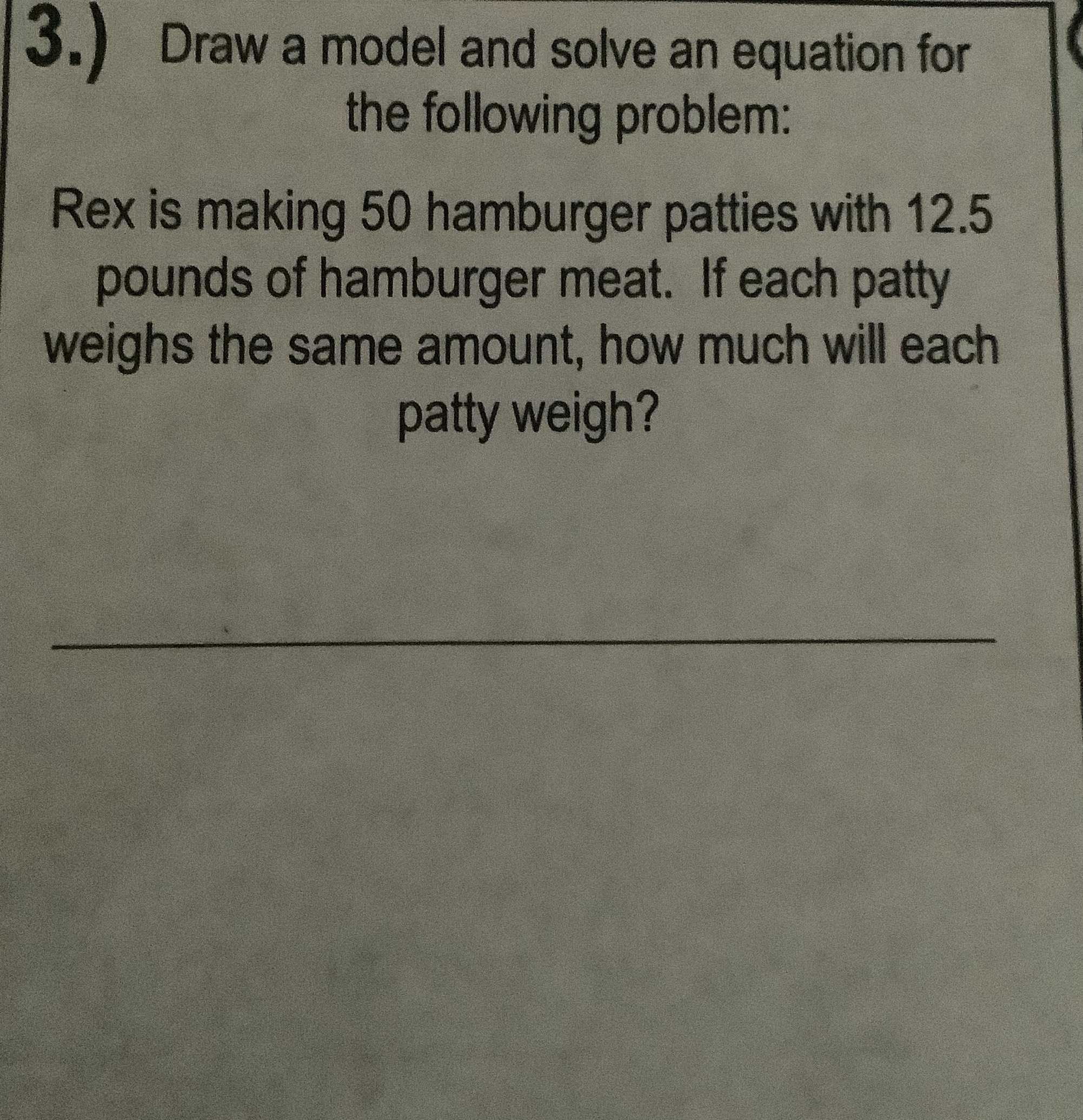### ¿Todavía tienes preguntas de matemáticas?

Pregunte a nuestros tutores expertos
Algebra
Pregunta3.) Draw a model and solve an equation for the following problem:

Rex is making $$50$$ hamburger patties with $$12.5$$ pounds of hamburger meat. If each patty weighs the same amount, how much will each patty weigh?